# Comparative advantage

(diff) ← Older revision | Latest revision (diff) | Newer revision → (diff)
Jump to: navigation, search

## Definition

The comparative advantage of an individual or a country is the thing that the individual or country can do with the maximum difference between the benefit and the opportunity cost. In other words, it is what the individual or country has the most advantage relative to other things the individual or country can do, and relative to things that others can do.

If individuals or countries choose to work according to their comparative advantage, then social utility (and hence, social surplus) is maximized. In principle, comparative advantage can emerge both in a market economy (through the effect of gains from trade) and in a centrally planned economy.

## Example

### A simple example involving two persons and two tasks

Suppose an economy has two individuals$A$ and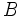$B$ and two activities$M$ and$N$. Further, suppose that:

•$A$ can generate$m_A$ units of worth per hour doing$M$ and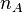$n_A$ units per hour doing$N$.
•$B$ can generate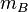$m_B$ units of worth per hour doing$M$ and$n_B$ units per hour doing$N$.

Suppose, further, that each person can choose to do exactly one of the two tasks, and they must do different tasks.

Since each can choose to do exactly one task, and they must do different tasks, there are two possible assignments:

•$A$ does$M$ and$B$ does$N$, yielding a total of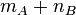$m_A + n_B$.
•$A$ does$N$ and$B$ does$M$, yielding a total of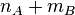$n_A + m_B$.

If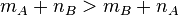$m_A + n_B > m_B + n_A$, then the first allocation is optimal, while if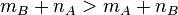$m_B + n_A > m_A + n_B$, the second allocation is optimal. What matters is the sign of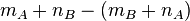$m_A + n_B - (m_B + n_A)$, which can also be viewed as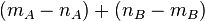$(m_A - n_A) + (n_B - m_B)$ (which is the sum of the gain over and above the opportunity cost of the two people for a particular choice they make) and it can also be viewed as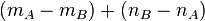$(m_A - m_B) + (n_B - n_A)$ (which is the sum of the individual competitive advantages).

Thus$A$ has a comparative advantage in$M$ if the following equivalent conditions are satisfied:

•$m_A + n_B > m_B + n_A$: Allocating$M$ to$A$ is the most efficient in terms of increasing output.
•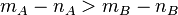$m_A - n_A > m_B - n_B$: In other words, the gain to$A$ over and above the opportunity cost for choosing$M$ over$N$ exceeds the gain to$B$.
•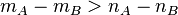$m_A - m_B > n_A - n_B$: In other words, the competitive advantage of$A$ in$M$ exceeds the competitive advantage of$A$ in$N$.

Note that it may well happen that$A$ is better than$B$ in both$M$ and$N$, but$A$ still has a comparative advantage only in$M$, because the difference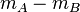$m_A - m_B$ exceeds the difference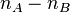$n_A - n_B$. Similarly$A$ may have a comparative advantage in$M$ despite$B$ being better at both.

### A more sophisticated example involving marginal costs and marginal utilities

In the previous example, we assumed that each person could choose to do exactly one task, and the two persons had to choose different tasks. If this constraint were absent, each person would simply do what he or she were best at, and the comparative advantage phenomenon may not arise.

Although a rigid limit on the number of people who can do each task may not exist in real life, there are a number of realistic features that could give rise to the same observations. These include:

• Increasing marginal cost of production (typically, in terms of some other factor of production) for each type of activity, that makes it worthwhile, after a certain point, to start doing another activity. For instance, there may be some land that is suitable for growing wheat and some land that is suitable for growing rice. Using land suitable for one crop to grow another is counterproductive. This leads to a tendency for some farmers to grow wheat and others to grow rice. Who chooses to grow which crop is then determined by comparative advantage. A similar case can be made when the capital stock for each type of productive activity is limited.
• Decreasing marginal utility: A society may gain a lot of utility from a bit of this and a bit of that, but less utility if only one type of good is produced. This has to do with the fact that the marginal utility from having more of a good is decreasing: the more there is, the less additional quantity of the good yields worth.

## Implementation of comparative advantage

### Comparative advantage in a market economy

In a market economy, where the goods produced are easy to trade, people tend to work in areas where they have comparative advantage, rather than what they are best at. This is because the loss they incur by not doing something they are more productive at is more than compensated by the gain they get from trading with somebody who is even better at that task.